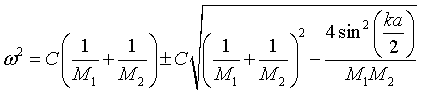## Phonon density of states for a 1-d linear chain with two masses

The phonon density of states can be calculated numerically from the dispersion relation,For this calculation, the frequency range from ω = 0 to the maximum frequency ωmax = √2C(1/M1 + 1/M2) is divided into 300 intervals. The frequencies are calculated for 10000 equally spaced values of k bewteen k = 0 and k = π/a. For each value of k there are two frequencies, one on the acoustic branch and one on the optical branch. The program counts how many times the frequency falls in each of the 300 frequency intervals to calculate the density of states.

 D(ω) [J-1 m-1] ω [1012 rad/s]
 Mass: M1 = [kg] Mass: M2 = [kg] Spring constant: C = [N/m]

 The density of states data on the right can be used to calculate the temperature dependence of thermodynamic quantities. Copy the data on the right and paste it into the programs that calculate the thermodynamic properties found by following the links below. ω [rad/s]    D(ω) [J-1 m-1]